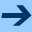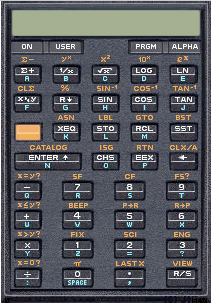﻿ Retro-FactorialBack to the Homepage of Factorial Algorithms. You might also whish to visit our FactorialCalculator page and see how today an ultrafast calculator works.RETRO-FACTORIAL

Once upon a time Hewlett-Packard build RPN-Calculators, like the legendary HP-41C.

Even today you can put an HP-41 on a Windows desktop and use it like in the good old days. The (virtual) Hardware by Warren Furlow and Software. And here you can find many other calculator emulators.

In 1977 Peter Henrici decscribed in Computational Analysis with the HP-25 Pocket Calculator a simple routine for calculating the Gamma function Gamma(x) = (x-1)!, x > 0, x real. Henrici used the Stirling formula tuned by the QD-algorithm (a Padé-approximation). With minor modifications this becomes the following code for the HP-41C.

 1 STO 02 + * 1 / x EXP 2 1 GTO 06 1 / x 1 2 3 STO 01 LBL 13 RCL 02 + PI 4 7 STO 02 4 1 / x * 5 RCL 02 6 * CHS RCL 02 6 LBL 06 * + RCL 02 / 7 x > y 1 / x RCL 02 LN SQRT 8 GTO 13 RCL 02 * + * 9 STO * 01 − 3 RCL 02 RCL 01 10 1 5 * * /

On my page comparing different approximation formulas for the factorial function I opted for Stieltjes' formula. So let us compare! The règles de jeu are: no more then 50 `HP-steps´ and only single-digit numbers. The formula used is discussed here. The HP-41C version is this sequence of keystrokes (the blue keystrokes are common to both routines):

 1 STO 02 + * LN RCL 02 2 1 GTO 06 1 / x 1 / 3 STO 01 LBL 13 RCL 02 − SQRT 4 7 STO 02 6 RCL 02 * 5 RCL 02 4 * * RCL 01 6 LBL 06 * + + / 7 x > y 1 / x 2 EXP 8 GTO 13 RCL 02 * 2 9 STO * 01 + 1 / x PI 10 1 5 RCL 02 *

Maybe a creative reader joins this friendly competition? (Please send me your solution.) Alternatively, you can use a very modern approach which combines object-oriented and functional programming (note the use of lambda expressions below) and use the cute little program below (written in C#), which simulates this evaluation of the factorial on the HP-41 calculator.

`   1:  using System;`
`   2:  using System.Linq;`
`   3:  using System.Collections.Generic;`
`   4:   `
`   5:  namespace RPNCalculator`
`   6:  {`
`   7:      public delegate void VoidFunc<T>(T arg);`
`   8:   `
`   9:      public static class FPSwitch`
`  10:      {`
`  11:          public static void Switch<T>`
`  12:          (`
`  13:              this IEnumerable<T> source,`
`  14:              Func<T, int> expr,`
`  15:              params VoidFunc<T>[] funcArray`
`  16:          )`
`  17:          {`
`  18:              foreach (T item in source)`
`  19:              {`
`  20:                  funcArray[expr(item)](item);`
`  21:              }`
`  22:          }`
`  23:      }`
`  24:   `
`  25:      public enum TokenType`
`  26:      {`
`  27:          Operand, Enter, Exchange,`
`  28:          Add, Subtract, Multiply, Divide, Invert,`
`  29:          Exp, Ln, Sqrt, PI`
`  30:      };`
`  31:   `
`  32:      public class Token`
`  33:      {`
`  34:          public TokenType Type;`
`  35:          public double Operand;`
`  36:   `
`  37:          public Token(TokenType type)`
`  38:          {`
`  39:              this.Type = type;`
`  40:          }`
`  41:   `
`  42:          public Token(double op)`
`  43:          {`
`  44:              this.Type = TokenType.Operand;`
`  45:              this.Operand = op;`
`  46:          }`
`  47:   `
`  48:          // The RPN Token Processor`
`  49:          public static double Processor(Token[] prog)`
`  50:          {`
`  51:              Stack<double> st = new Stack<double>();`
`  52:   `
`  53:              prog.Switch`
`  54:              (`
`  55:                  i => (int)i.Type,`
`  56:                  s => st.Push(s.Operand),`
`  57:                  s => st.Push(st.Peek()),`
`  58:                  s =>`
`  59:                  {`
`  60:                      double a = st.Pop(), b = st.Pop();`
`  61:                      st.Push(a); st.Push(b);`
`  62:                  },`
`  63:                  s => st.Push(st.Pop() + st.Pop()),`
`  64:                  s => st.Push(-st.Pop() + st.Pop()),`
`  65:                  s => st.Push(st.Pop() * st.Pop()),`
`  66:                  s => st.Push(1 / st.Pop() * st.Pop()),`
`  67:                  s => st.Push(1 / st.Pop()),`
`  68:                  s => st.Push(Math.Exp(st.Pop())),`
`  69:                  s => st.Push(Math.Log(st.Pop())),`
`  70:                  s => st.Push(Math.Sqrt(st.Pop())),`
`  71:                  s => st.Push(Math.PI)`
`  72:              );`
`  73:   `
`  74:              return st.Pop();`
`  75:          }`
`  76:      }`

That's all! Now we can start writing programs for this HP-41 simulator. Here is the RETRO-FACTORIAL program:

`  77:      class Factorial`
`  78:      {`
`  79:          static double factorial(double x)`
`  80:          {`
`  81:              Token[] program = `
`  82:              {`
`  83:                  new Token(x),`
`  84:                  new Token(1),`
`  85:                  new Token(TokenType.Add),`
`  86:                  new Token(TokenType.Enter),`
`  87:                  new Token(TokenType.Enter),`
`  88:                  new Token(TokenType.Enter),`
`  89:                  new Token(TokenType.Enter),`
`  90:                  new Token(4),`
`  91:                  new Token(TokenType.Multiply),`
`  92:                  new Token(TokenType.Invert),`
`  93:                  new Token(TokenType.Add),`
`  94:                  new Token(5),`
`  95:                  new Token(TokenType.Multiply),`
`  96:                  new Token(TokenType.Invert),`
`  97:                  new Token(TokenType.Exchange),`
`  98:                  new Token(6),`
`  99:                  new Token(TokenType.Multiply),`
` 100:                  new Token(TokenType.Add),`
` 101:                  new Token(2),`
` 102:                  new Token(TokenType.Multiply),`
` 103:                  new Token(TokenType.Invert),`
` 104:                  new Token(TokenType.Exchange),`
` 105:                  new Token(TokenType.Enter),`
` 106:                  new Token(TokenType.Ln),`
` 107:                  new Token(1),`
` 108:                  new Token(TokenType.Subtract),`
` 109:                  new Token(TokenType.Multiply),`
` 110:                  new Token(TokenType.Add),`
` 111:                  new Token(TokenType.Exp),`
` 112:                  new Token(TokenType.Exchange),`
` 113:                  new Token(TokenType.PI),`
` 114:                  new Token(2),`
` 115:                  new Token(TokenType.Multiply),`
` 116:                  new Token(TokenType.Exchange),`
` 117:                  new Token(TokenType.Divide),`
` 118:                  new Token(TokenType.Sqrt),`
` 119:                  new Token(TokenType.Multiply)`
` 120:              };`
` 121:   `
` 122:              return Token.Processor(program);`
` 123:          }`
` 124:   `
` 125:          static void Main()`
` 126:          {`
` 127:              for (int i = 0; i < 12; i++)`
` 128:              {`
` 129:                  Console.WriteLine(i + " -> " + factorial(i));`
` 130:              }`
` 131:          }`
` 132:      }`
` 133:  }`Back to the Homepage of Factorial Algorithms. You might also whish to visit our FactorialCalculator page and see how today an ultrafast calculator works.